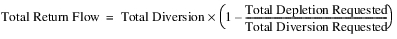Dispatch Methods
The Power Plant Diversion solves given either diversion requests and available water (most common) or just diversion. There are no user methods for this object.Solve given Diversion Requested
This dispatch method will solve when diversion requests and the available water are known. It computes the total diversion and depletion (consumption).
The Total Diversion Requested is compared with the Total Available Water slot. If the total request can be met, the Total Diversion slot is set to that amount; if not, the Total Diversion slot is set to the Total Available Water amount. If a total deficit occurs in the Diversion, the Return Flows are recalculated based on the ratio of the Total Depletion Requested to the Total Diversion Requested. The Total Depletion Shortage is computed as the difference between Total Depletion Requested and Depletion.
Required Known Slots
Required Unknown Slots
Method Details
Following is a general description of the interaction among the Power Plant Diversion, Generators, and Reach/Reservoir.
1. Reach/Reservoir Available For Diversion is set.
2. This value propagates to Power Plant Diversion: Total Available Water.
3. Solve given Diversion Requested dispatches on the Power Plant Diversion object.
4. Within the dispatch method, Total Diversion and Total Return Flow are calculated.
5. The values propagate to the reach or reservoir and that object re-solves.
The Solve given Diversion Requested performs as follows.
1. If Total Depletion Requested is not specified, it is set equal to the Total Diversion Requested.
2. If Total Diversion Requested is greater than or equal to the Total Available Water:
Total Diversion = Total Available Water3. If the Total Diversion Requested is less than Total Available Water:
– Total Diversion = Total Diversion Requested
– Total Return Flow = Total Diversion - Total Depletion Requested
4. Total Depletion is set as the difference between Total Diversion and Total Return Flow.
5. Total Depletion Shortage is computed as Total Depletion Requested minus Total Depletion.
6. Total Diversion Shortage is computed as Total Diversion Requested minus Total Diversion.Solve given Diversion
This dispatch method will solve when diversion is known. It computes the total return flow and depletion (consumption).
Required Known Slots
Required Unknown Slots
Method Details
The Solve given Diversion dispatch method performs as follows.
1. If Total Diversion Requested is not specified, assume it is equal to Total Diversion. The slot is not set, however.
2. If Total Depletion Requested is not specified, assume it is equal to the Total Diversion Requested, if valid. Otherwise assume it is equal to Total Diversion. The slot is not set, however.
3. If Total Diversion Requested is zero, set Total Return flow equal to Total Diversion minus Total Depletion Requested.
4. Else, if Total Diversion is less than Total Diversion Requested, set the following:5. Otherwise, Total Diversion is greater than Total Diversion Requested. Set Total Return Flow equal to Total Diversion minus Total Depletion Requested.
6. Then, Total Depletion is set as the difference between Total Diversion and Total Return Flow.
7. Total Depletion Shortage is computed as Total Depletion Requested minus Total Depletion.
8. Total Diversion Shortage is computed as Total Diversion Requested minus Total Diversion.

Revised: 01/11/2023